International Journal of Applied Mathematics and Theoretical Physics
Volume 3, Issue 1, January 2017, Pages: 14-19

Methodology Article

Exemplification of Dimensional Analysis via MATLAB® Using Eigen Values

Kamal Isa Masoud Al-Malah

Department of Chemical Engineering, Higher Colleges of Technology, Ruwais, UAE

Email address: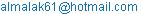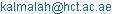To cite this article:

Kamal Isa Masoud Al-Malah. Exemplification of Dimensional Analysis via MATLAB® Using Eigen Values. International Journal of Applied Mathematics and Theoretical Physics. Vol. 3, No. 1, 2017, pp. 14-19. doi: 10.11648/j.ijamtp.20170301.13

Received: October 15, 2016; Accepted: November 2, 2016; Published: January 18, 2017

Abstract: In this research article, a set of dimensional physical quantities is transformed into a dimensionless group (or ratio). For a given set of dimensional variables, the physical variables represent the rows and their dimensions represent the columns of a dimensions-matrix. The dimensions-matrix is rearranged both column- and row-wise. The columns are sorted in ascending order based on the column sum and then on the largest negative entry (i.e., cell value). On the other hand, the rows are sorted in descending order based on the number of non-zero entries found in each row and then on the higher first entry. With the aid of MATLAB®, it was found that the proposed method leads to a permutation matrix that has an Eigen vector whose elements represent the exponent for each physical dimensional quantity such that, at the end, a dimensionless group (or ratio) can be formulated, like Schmidt, Nusselt, Reynolds, and Peclet number. The method, however, was found to work well with a set of physical quantities where each is raised to an exponent of ±1.

Keywords: Dimensional Analysis, Reynolds, Nusselt, Schmidt, Peclet, Froude, Exemplification, Chemical Engineering Education, MATLAB, Eigen Vector

Contents

1. Introduction

In the first example, the physics behind models underlying mass transfer was portrayed for both gas and liquid world . In the second example, the demarcation line between a finite and semi-infinite medium was made clear and the applications of the latter in real chemical engineering life were also demonstrated . In the third example, simple yet reliable models to predict some physical properties of a hydrocarbon were introduced [3, 4]. In this methodological article, a computational approach will be presented to generate a dimensionless group (or ratio) as a function of some given physical dimensional quantities using the matrix approach. MATLAB® will be utilized to find the Eigen vector associated with the generated permutation matrix for the given dimensions-matrix.

In engineering, applied mathematics, and physics, dimensional analysis is a technique that is used to analyze the relationships among different physical quantities by identifying their fundamental dimensions (such as length, mass, time, energy, power, temperature, and electric charge) and rearranging such dimensional physical quantities into a lump sum in the form of a dimensionless group or ratio. In general, such a technique is mainly used as a tool for obtaining information about a physical system that is complicated enough for a full mathematical solution to be feasible. For instance, the generated dimensionless groups serve as scale-independent quantitative indicators for characterizing the extent or intensity of phenomena occurring in systems that involve transport phenomena and chemical reactions, as well.

The Buckingham π theorem [5, 6] is a key theorem in dimensional analysis. In simple terms, it states that an equation involving n number of physical variables which are expressible in terms of k independent fundamental physical quantities can be expressed in terms of p = n - k dimensionless parameters.

Leona and Zhub  presented a dimensional analysis for determining the optimal discharge and penstock diameter in impulse and reaction turbines. They derived various dimensionless relationships between power production, flow discharge, and head losses. Those relationships were used to withdraw general insights on determining optimal discharge and optimal penstock diameter.

In the past, dimensional analysis was applied mostly to experimental research. Now, application of the analysis to model equations has become more important. Equations reformulated with the use of dimensional analysis are usually clearer and easier to handle computationally.

Matuszak  demonstrated the principles of dimensional analysis on the problem of the second moment of area for a rectangle. The transformation of equations to unit-independent form was discussed on the example of concrete constitutive equations. The equation that related tensile and compressive strength of concrete was also analyzed. He suggested that any equation that represents a universal law should be independent of system of units. In his opinion, finding the universal law is often too difficult, but an equation could still be written in the form that is independent of units. Difficulties with finding such a form of equation may suggest model incorrectness or insufficient problem knowledge and may suggest some future research directions.

Olmos et al.  studied the experimental characterization of particle suspension in orbital shakers. They performed a dimensional analysis approach, which led them to a correlation relating a Froude number (in which the critical agitation for a complete particle suspension is embedded) to four other dimensionless numbers. Their findings revealed that high orbital shaking diameters and large cylindrical vessels should be promoted to get micro-carriers into suspension at a minimized power dissipation per unit volume.

Sollund et al.  examined the problem of an axially loaded pipeline resting on semi-infinite elastic supports on either side of a free span. A dimensional analysis of the problem was carried out, and it was demonstrated that the Eigen-frequencies and associated maximum modal stresses of the pipe could be uniquely represented as functions of only two non-dimensional parameters. Explicit analytical formulae for the modal response quantities were derived, with an improved accuracy and applicability range as compared to approximate expressions that were widely used in the pipeline industry.

Finally, for further details on MATLAB® applications, please refer to .

2. Methodology

The MATLAB®-based approach will be demonstrated here for the sake of generating a dimensionless ratio out of a set of dimensional physical variables via presenting different examples. It is worth-mentioning here that the approach was concluded after an exhaustive inspection of all possible permutations both row-wise and column-wise for a given set of physical variables (i.e., rows) with known dimensions (i.e., columns). For each permutation, the corresponding Eigen vector was calculated to see the best solution that gives a successful matching between the Eigen values and the physical quantities under study. Consequently, the postulated method was concluded after many failing scenarios.

2.1. Example 1: Schmidt Number

Consider the following physical properties: The density of a fluid, ρ, its viscosity, μ, and its diffusivity, D. Table 1 shows the dimensions of each physical quantity.

Table 1. The dimensions matrix for physical quantities as a function of length, mass, and time.

 Length [m] Mass [kg] Time [s] # of non-zero entries ρ -3 1 0 2 μ -1 1 -1 3 D 2 0 -1 2 Column Sum -2 2 -2

For the sake of implementing the proposed method, I will pinpoint the major steps:

2.1.1. The First Step: Column Sorting

The columns will be sorted in ascending order based on the column sum. If two column sums are equal, then the column with the largest negative entry (i.e., cell value) will be allocated first. For example, the sum of "Length" column is: -3-1+2=-2. On the other hand, the sum of "Time" column is: 0-1-1=-2. Since the value of the column sum is the same, then we will assign the column with the largest negative entry as the first column, which will be in this case "Length" column because it has -3 as the largest negative value. The second column will then be reserved for "Time", and finally the third column will be left for "Mass".

Therefore, the ascending order of columns will be: "Length", "Time", and "Mass" column.

Table 2 shows the new ascending order of columns as far as the column sum is concerned.

Table 2. Sorting columns in ascending order based on the column sum and if the two sums are equal we will go by the largest negative entry (or cell value).

 Length [m] Time [s] Mass [kg] # of non-zero entries ρ -3 0 1 2 μ -1 -1 1 3 D 2 -1 0 2 Column Sum -2 -2 2

2.1.2. The Second Step: Row Sorting

The rows will be sorted in descending-order based on the number of non-zero entries (i.e., cell values) found in each row. If the number of entries is the same for two rows, then the row with the higher first entry will be taken first. From Table 2, ("μ") row has three non-zero entries; hence, it should be assigned first. ("ρ") row and ("D") row both have two non-zero entries; hence, we will reserve the second row for ("D") as it has the higher first entry (i.e., 2>-3) and finally the third row will be left for ("ρ"), as shown in Table 3.

Table 3. Sorting rows in descending order based on the number of non-zero entries and if the two numbers are equal we will go by the higher first entry.

 Length [m] Time [s] Mass [kg] # of non-zero entries μ -1 -1 1 3 D 2 -1 0 2 ρ -3 0 1 2 Column Sum -2 -2 2

2.1.3. The Third Step: Defining the Coefficient Matrix

Let us define ASc as 3×3 matrix: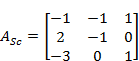(1)

2.1.4. The Fourth Step: Finding the Permutation Matrix, PSc

>>[LSc, USc, PSc] = lu(ASc);

This MATLAB® built-in function returns the unit lower triangular matrix LSc, the upper triangular matrix USc, and the permutation matrix PSc such that PSc*ASc = LSc*USc. For example, the permutation matrix, PSc, for ASc matrix, will be:(2)

2.1.5. The Fifth Step: Finding Eigen Values for the Permutation Matrix

>>EVSc = eig(PSc)

This MATLAB® built-in function produces a column vector E containing the eigenvalues of the square matrix PSc.

>> EVSc=eig(PSc)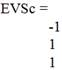2.1.6. The Sixth Step: Formulating the Dimensionless Ratio (or Number)

The dimensionless group will be: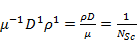(3)

This basically represents the reciprocal value of Schmidt number in mass transfer, where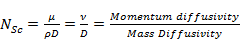.

2.2. Example 2: Nusselt Number

Consider the following physical properties: The heat transfer coefficient of a fluid, h, its thermal conductivity, κ, and some characteristic length, L. Table 4 shows the dimensions of each physical quantity.

Table 4. The dimensions matrix for physical quantities as a function of power, length, and temperature.

 Power [W] Length [m] Temperature [°C] # of non-zero entries h 1 -2 -1 3 κ 1 -1 -1 3 L 0 1 0 1 Column Sum 2 -2 -2

The procedure, outlined in Example 1, will be traced a step by step but extra details will be gradually removed.

2.2.1. The First Step: Column Sorting

The columns will be sorted in ascending order based on the column sum. The ascending order of columns will be: "Length", "Temperature", and "Power" column.

Table 5 shows the new ascending order of columns as far as the column sum is concerned.

Table 5. Sorting columns in ascending order based on the column sum and if the sums are equal we will go by the largest negative entry (or cell value).

 Length [m] Temperature [°C] Power [W] # of non-zero entries h -2 -1 1 3 κ -1 -1 1 3 L 1 0 0 1 Column Sum -2 -2 2

2.2.2. The Second Step: Row Sorting

The rows will be sorted in descending-order based on the number of non-zero entries (i.e., cell values) found in each row. From Table 5, ("h") row and ("κ") row both have equal number of non-zero entries; hence, we will reserve the first row for "κ" as it has the higher first entry (i.e., -1>-2) followed by "h" row, and finally the third row will be left for "L", as shown in Table 6.

Table 6. Sorting rows in descending order based on the number of non-zero entries and if the two numbers are equal we will go by the higher first entry.

 Length [m] Temperature [°C] Power [W] # of non-zero entries κ -1 -1 1 3 h -2 -1 1 3 L 1 0 0 1 Column Sum -2 -2 2

2.2.3. The Third Step: Defining the Coefficient Matrix

Let us define ANu as 3×3 matrix: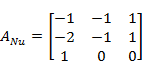(4)

2.2.4. The Fourth Step: Finding the Permutation Matrix, PNu

>>[LNu, UNu, PNu] = lu(ANu);

The permutation matrix, PNu, for ANu matrix, will be:(5)

2.2.5. The Fifth Step: Finding Eigen Values for the Permutation Matrix

The column vector EVNu, containing the eigenvalues of the square matrix PNu, is given by:

>> EVNu=eig(PNu)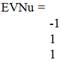2.2.6. The Sixth Step: Formulating the Dimensionless Ratio (or Number)

The dimensionless group will be: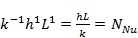(6)

This basically represents the Nusselt number in heat transfer,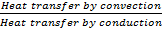.

2.3. Example 3: Reynolds Number

Consider the following physical properties: The density of a fluid, ρ, its viscosity, μ, its velocity, u, and pipe diameter, d. Table 7 shows the dimensions of each physical quantity.

Table 7. The dimensions matrix for physical quantities as a function of length, mass, and time.

 Length [m] Mass [kg] Time [s] # of non-zero entries ρ -3 1 0 2 μ -1 1 -1 3 d 1 0 0 1 u 1 0 -1 2 Column Sum -2 2 -2

2.3.1. The First Step: Column Sorting

Table 8 shows the new ascending order of columns as far as the column sum is concerned.

Table 8. Sorting columns in ascending order based on the column sum and if the sums are equal we will go by the largest negative entry (or cell value).

 Length [m] Time [s] Mass [kg] # of non-zero entries ρ -3 0 1 2 μ -1 -1 1 3 d 1 0 0 1 u 1 -1 0 2 Column Sum -2 -2 2

2.3.2. The Second Step: Row Sorting

Table 9 shows rows in the descending order.

Table 9. Sorting rows in descending order based on the number of non-zero entries and if the two numbers are equal we will go by the higher first entry.

 Length [m] Time [s] Mass [kg] # of non-zero entries μ -1 -1 1 3 u 1 -1 0 2 ρ -3 0 1 2 d 1 0 0 1 Column Sum -2 -2 2

2.3.3. The Third Step: Defining the Coefficient Matrix

Let us define ARe as 4×3 matrix: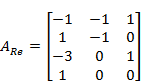(7)

2.3.4. The Fourth Step: Finding the Permutation Matrix, PRe

The permutation matrix, PRe, for ARe matrix, will be: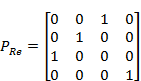(8)

2.3.5. The Fifth Step: Finding Eigen Values for the Permutation Matrix

The column vector EVRe, containing the eigenvalues of the square matrix Pre, is given by:

>> EVRe=eig(PRe)2.3.6. The Sixth Step: Formulating the Dimensionless Ratio (or Number)

The dimensionless group will be: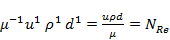(9)

This basically represents Reynolds number in fluid mechanics, where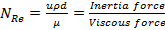.

2.4. Example 4: Peclet Number

Consider the following physical properties: The density of a fluid, ρ, its velocity, u, its thermal conductivity, κ, its heat capacity, Cp, and pipe diameter, d. Table 10 shows the dimensions of each physical quantity.

Table 10. The dimensions matrix for physical quantities as a function of length, mass, time, and temperature.

 Length [m] Mass [kg] Time [s] Temperature [°C] # of non-zero entries ρ -3 1 0 0 2 Cp 2 0 -2 -1 3 κ 1 1 -3 -1 4 u 1 0 -1 0 2 d 1 0 0 0 1 Column Sum 2 2 -6 -2

2.4.1. The First Step: Column Sorting

Table 11 shows the new ascending order of columns as far as the column sum is concerned.

Table 11. Sorting columns in ascending order based on the column sum and if the sums are equal we will go by the largest negative entry (or cell value).

 Time [s] Temperature [°C] Length [m] Mass [kg] # of non-zero entries ρ 0 0 -3 1 2 Cp -2 -1 2 0 3 κ -3 -1 1 1 4 u -1 0 1 0 2 d 0 0 1 0 1 Column Sum -6 -2 2 2

2.4.2. The Second Step: Row Sorting

Table 12 shows rows in the descending order.

Table 12. Sorting rows in descending order based on the number of non-zero entries and if the two numbers are equal we will go by the higher first entry.

 Time [s] Temperature [°C] Length [m] Mass [kg] # of non-zero entries κ -3 -1 1 1 4 Cp -2 -1 2 0 3 ρ 0 0 -3 1 2 u -1 0 1 0 2 d 0 0 1 0 1 Column Sum -6 -2 2 2

2.4.3. The Third Step: Defining the Coefficient Matrix

Let us define APe as 4×3 matrix: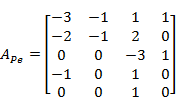(10)

2.4.4. The Fourth Step: Finding the Permutation Matrix, PPe

The permutation matrix, PPe, for APe matrix, will be:(11)

2.4.5. The Fifth Step: Finding Eigen Values for the Permutation Matrix

The column vector EVPe, containing the eigenvalues of the square matrix PPe, is given by:

>> EVPe=eig(PPe)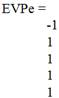2.4.6. The Sixth Step: Formulating the Dimensionless Ratio (or Number)

The dimensionless group will be: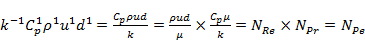(12)

This basically represents Peclet number in heat transfer, where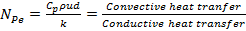.

2.5. Example 5: Froude Number

Consider the following physical properties: The velocity of an object, u, its length, L, and the gravity constant, g. Table 13 shows the dimensions of each physical quantity.

Table 13. The dimensions matrix for physical quantities as a function of length, mass, and time.

 Length [m] Time [s] # of non-zero entries ν 1 -1 2 g 1 -2 2 L 1 0 1 Column Sum 3 -3

2.5.1. The First Step: Column Sorting

Table 14 shows the new ascending order of columns as far as the column sum is concerned.

Table 14. Sorting columns in ascending order based on the column sum and if the two sums are equal we will go by the largest negative entry (or cell value).

 Time [s] Length [m] # of non-zero entries ν -1 1 2 g -2 1 2 L 0 1 1 Column Sum -3 3

2.5.2. The Second Step: Row Sorting

The rows will be sorted in descending-order, as shown in as shown in Table 15.

Table 15. Sorting rows in descending order based on the number of non-zero entries and if the two numbers are equal we will go by the higher first entry.

 Time [s] Length [m] # of non-zero entries ν -1 1 2 g -2 1 2 L 0 1 1 Column Sum -3 3

2.5.3. The Third Step: Defining the Coefficient Matrix

Let us define AFr as 3×2 matrix: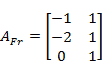(13)

2.5.4. The Fourth Step: Finding the Permutation Matrix, PFr

The permutation matrix, PFr, for AFr matrix, will be: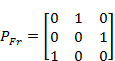(14)

2.5.5. The Fifth Step: Finding Eigen Values for the Permutation Matrix

The column vector EVFr, containing the eigenvalues of the square matrix PFr, is given by:

>> EVFr=eig(PFr)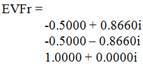2.5.6. The Sixth Step: Formulating the Dimensionless Ratio (or Number)

Here, I would say that the approach did not work as expected to generate the dimensionless Froude number: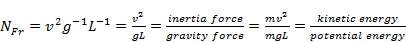(15)

3. Conclusion

In brief, the proposed method is appealing and promising as it successfully hooks the power of each physical quantity with an Eigen-value out of the Eigen-vector found for the generated permutation matrix. The permutation matrix is basically a cosmetically treated dimensions-matrix that is subject to both column and row operation. The method, however, was found to work well with a set of physical quantities where each is raised to an exponent of ±1.

Hopefully, this method will open up the doors for future work in this direction; in particular, how to reproduce more than one dimensionless ratio for a given set of dimensional physical quantities, or when a physical quantity is raised to a power other than ±1.

References

1. Al-Malah, K."Exemplification of the Concept of Diffusivity in Mass Transfer", International Journal of Chemical Engineering and Applied Sciences, 2(4): 24-26 (2012). http://urpjournals.com/tocjnls/9_12v2i4_1.pdf.
2. Al-Malah, K. "Exemplification of a Semi-Infinite vs. Finite Medium in Heat/Mass Transfer". Chemical Engineering & Process Techniques, 2(1): 1021 (2014). http://www.jscimedcentral.com/ChemicalEngineering/chemicalengineering-2-1021.pdf
3. Al-Malah, K."Prediction of Normal Boiling Points of Hydrocarbons Using Simple Molecular Properties", J. of Advanced Chemical Engineering, Vol. 3 (2013). doi:10.4303/jace/235654. http://www.ashdin.com/journals/JACE/235654.pdf.
4. Al-Malah, Kamal I. "RK-, SRK-, & SRK-PR-Type Equation of State for Hydrocarbons, Based on Simple Molecular Properties". Journal of Applied Chemical Science International, 2(2): 65-74, (2015). http://www.ikpress.org/issue.php?iid=451&id=35.
5. Buckingham, E. "On Physically Similar Systems: Illustrations of the Use of Dimensional Equations." Phys. Rev. 4: 345-376, (1914).
6. Buckingham, E. "Model Experiments and the Form of Empirical Equations." Trans. ASME 37: 263, (1915).
7. Leona, A.S. and L. Zhub. "A dimensional analysis for determining optimal discharge and penstock diameter in impulse and reaction water turbines", Renewable Energy, 71: 609–615, (2014). doi:10.1016/j.renene.2014.06.024.
8. Matuszak, A. "Dimensional Analysis can Improve Equations of the Model". Procedia Engineering, 108: 526-535, (2015). doi:10.1016/j.proeng.2015.06.174.
9. Olmos, E., K. Loubiere, C. Martin, G. Delaplace, and Annie Marc. "Critical agitation for microcarrier suspension in orbital shaken bioreactors: Experimental study and dimensional analysis", Chemical Engineering Science, 122: 545–554, (2015). doi:10.1016/j.ces.2014.08.063.
10. Sollund, H.A., K. Vedeld, and O. Fyrileiv. "Modal response of free spanning pipelines based on dimensional analysis", Applied Ocean Research, 50: 13-29, (2015). doi:10.1016/j.apor.2014.12.001.
11. Al-Malah, K., "MATLAB®: Numerical Methods with Chemical Engineering Applications", McGraw Hill, Inc., New York, USA.ISBN: 9780071831284 (2013).http://www.mhprofessional.com/product.php?isbn=0071831282

 Contents 1. 2. 2.1. 2.2. 2.3. 2.4. 2.5. 3.
Article ToolsAbstractPDF(235K)
Follow on us
PUBLICATION SERVICE
JOIN US
RESOURCES
SPECIAL SERVICES
ADDRESS
Science Publishing Group
548 FASHION AVENUE
NEW YORK, NY 10018
U.S.A.
Tel: (001)347-688-8931